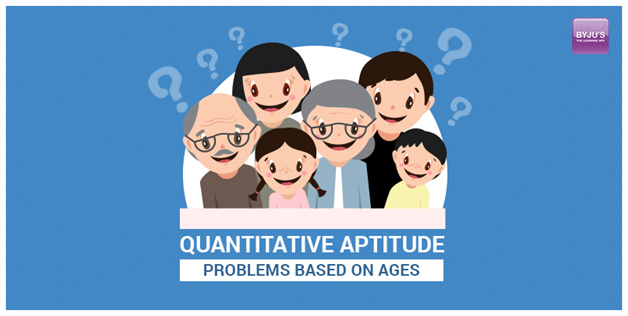### Article Post by ByjusAmong all the easy topics in quantitative aptitude section, Problems based on ages is one of the scoring and easy topics if you can crack the question and understand it in a simple way. This topic basically requires basic knowledge of Linear Equation and ratio and halfway you are done there. There are many formulas in this topic but if you are strong at the above mention two topics you can solve any question related to ages.

The only reason student are not able to solve these problems is because they usually are unable to frame the equations or unable to express the data given in a proper manner. Let’s discuss and solve some of the most asked questions by students to give a more clear view towards this topic.

1. The ratio of present ages of M,N and O is 4:7:9, before eight years, sum of M,N and O ages was 56. How old are they now (in years)?

Solution: Let ratio of present ages of M,N and O is 4x:7x:9x

And sum of the ages before eight years = 56

(4x – 8) + (7x – 8) + (9x – 8) = 56

20x = 80

x = 4

A = 4x = 16 years

B = 7x = 28 years

C = 9x = 36 years

2. The sum of ages of Gopal and Rinku is 40 years. 5 years from now the ratio will be 7:3. Find Rinku’s age?

Solution: Given 5yrs from now ratio will be 7:3

Therefore 5yrs from now, age of Gopal = 7X and Rinku = 3X

So, present age of Gopal = 7x – 5 and Rinku = 3X – 5

Sum of present ages is = 40

(3X – 5) + (7X – 5) = 40

10X = 50

X = 5

Therefore present Rinku‘s age = 3X – 5 = 10yrs

3. Kalpana’s present age is one-fourth of her Uncle. 6 years from now Uncle’s age will be twice the Raghav’s age. If Raghav celebrated his fifth birthday, 8 years ago. What is the present age of Kalpana?

Solution: Let present age of Kalpana is X, then Uncle’s age = 4X

6 years hence,

Uncle’s age = 4X + 6

2 (Age of Raghav) = 4X + 6

Age of Raghav = 2X + 3

Present age of Raghav = 2X + 3 – 6 = 2X – 3

Raghav celebrated his 5th birthday 8 years ago

So, Present age of Raghav is 5 + 8 = 13 years

2X – 3 = 13

2X = 16

X = 8 years.

Shortcut:

Raghav celebrated his 5th birthday 8 years ago.

Present age of Raghav = 13

After 6years, Uncle’s age will be twice of the Raghav.

2x(13 + 6) = 4X + 6

X = 8 years

4. A boy’s age is 133(1/3)% of what it was 8 years ago, but 80% of what it will be after 8 years. What is his present age?

Solution: Let the present age be X years.

Then 133(1/3)% of (X – 8) = X and 80%(X + 8) = X

So, 133(1/3)% of (X – 8) = 80%(X + 8)

4(X – 8)/3 = 4(X + 8)/5

5(X – 8) = 3(X + 8)

2X = 64

X = 32

Shortcut:

Solve any equation to get the answer.

133(1/3)% of (X – 8) = X

4(X – 8)/3 = X

4X – 32 = 3X

X = 32 years.

These are a few basic examples that usually students find difficult to solve problems related to ages. Hope that you get benefited with this discussion and may you do well in all your Bank Exams, Good Luck!